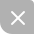# 一个Java小白通向数据结构算法之旅(7) 简单排序总结

###前言

###几种简单排序的比较

• 一般情况几乎不太使用冒泡排序算法，它过于简单了。当数据量很小的时候，它会有些应用价值。

• 选择排序虽然把交换次数降到了最低，但比较的次数仍然很大。当数据量很小，并且交换次数相当于比较数据更加耗时的情况下，可以应用选择排序。

• 但数据量比较小或基本上有序时，插入排序算法是三种简单排序算法中最好的选择。

###归纳

• 这几种简单排序算法中都假定了数据作为数据存储结构。

• 排序包括比较数组中数据项的关键字和移动相应的数据项，直到它们排好序为止。

• 3种简单排序算法复杂度都是 O(N^2) ,不过插入排序在某种情况下会比冒泡排序，选择排序快的多。算法复杂度结果为10^8 的算法是秒级算法。O(N^2)的算法，在数据项小于10000时，运行才会是秒级。

• 不变性是指算法运行时保持不变的条件。冒泡排序的不变性是out右边的所有数据项为有序。选择排序中不变性是下标小于或等于out的数据都是有序的。插入排序中的不变性是下标比out小的数据项是局部有序的。这里out指的是循环中的变量。

• 冒泡排序是效率最差的算法，但它是最简单的。

• 插入排序算法是O(N^2)排序算法中应用最多的。

• 如果具有相同关键字的数据项，经过排序他们的顺序保持不变，这样的排序就是稳定的。

• 这几种排序中，除了需要初始数组之外，还需要一个临时变量。

###对象排序 我们有时候需要比较对象中的关键字，来给一组对象进行排序。

• 先定义一个Person类。
public class Person {
private String name;
private Integer age;
private Integer height;

public Person(String name, Integer age, Integer height) {
super();
this.name = name;
this.age = age;
this.height = height;
}

public String getName() {
return name;
}

public void setName(String name) {
this.name = name;
}

public Integer getAge() {
return age;
}

public void setAge(Integer age) {
this.age = age;
}

public Integer getHeight() {
return height;
}

public void setHeight(Integer height) {
this.height = height;
}

public void displayPerson() {
System.out.println("name:" + name + ", age:" + age + ", height:" + height);
}
}

• 再去定义一个ArrayInOb数组类，我们是用以Person类的name属性为关键字去给Person类型的数组去排序，通过String类中的compareTo()方法按字典顺序去比较字符串，首先会比较出2个字符串长度。

• 在较短的字符串长度之内，去比较两者每一个位置上的字符是否相等。如果不相等，则直接返回该位置上字符的ASCII码之差，假如在第0号位置上字符相等的话，那么就去比较第1号位置上，以此类推。如果每个位置上字符的ASCII码都相等的话，那么返回2个字符串长度之差。

public class ArrayInOb {
private Person[] a;
private int size;

public ArrayInOb(int max) {
a = new Person[max];
size = 0;
}

public void insert(String name, Integer age, Integer height) {
a[size] = new Person(name, age, height);
size++;
}

public void display() {
for (int j = 0; j < size; j++) {
a[j].displayPerson();
}
System.out.println("");
}

public void insertSort() {
for (int i = 0; i < size; i++) {
Person temp = a[i];
int j = i - 1;

while (j >= 0 && a[j].getName().compareTo(temp.getName()) > 0) {
a[j + 1] = a[j];
j--;
}

a[j + 1] = temp;
}
}
}

• 编写测试Demo
public class ObjectSortDemo {

public static void main(String[] args) {
int maxSize = 100;
ArrayInOb arrayInOb = new ArrayInOb(maxSize);

arrayInOb.insert("garrett", 18, 173);
arrayInOb.insert("cmazxiaoma", 21, 170);
arrayInOb.insert("xiaodingding", 22, 165);
arrayInOb.insert("xiaoma", 23, 155);

System.out.println("befor sorting");
arrayInOb.display();
arrayInOb.insertSort();

System.out.println("After sorting");
arrayInOb.display();

String a = "xiaodingding";
String b = "xiaoma";

System.out.println(a.compareTo(b));
}
}

• 运行，输出结果。很明显看到一组Person对象是按name属性去排序，从小到大。另外"xiaodingding""xiaoma"2个字符串比较，在比较第0 - 3个元素时，也就是"xiao"这个4字符时，它们都相等。可是比较到第4个元素时，也就是"d""m"时。“d”ASCII码是100“m”ASCII码是109。它们ASCII码之间差值是-9，调用compareTo()结果也就是-9。所以”xiaodingding”要排在”xiaoma”的前面。

###关于插入排序的题目 题目是这样的。有一个有序的方法用来删除数组中相同的数据项，要求使用插入排序。

#####解题思路

• 插入排序算法中用一个循环嵌套算法，将数组中的每一个数据项与其他数据项一一比较。

• 当找到一个重复数据项的时候，通常用一个小于任何值的关键字才改写这个相同项(如果所有值都是正数，那么可以取-1)。

• 一般的插入排序算法就会像处理其他数据项一样，来处理这个修改了关键值的数据项，把它移动下标为0的位置。从现在开始，算法可以忽略这个数据项，下一个相同的数据项将被移动到下标为1的位置，以此类推。

• 排序完成之后，所有相同的数据项(关键字为-1)都在数组的开头部分，可以改变数组的容量并把需要的数据前移到数组下标为0的位置。

#####手写代码

public class InsertSortDemo {

public static int a[] = new int[] { 11, 20, 5, 5, 1, 1, 2, 10, 20, 9 };

public static void main(String[] args) {
insertSortDeleteRepeatElement();
}

public static void insertSortDeleteRepeatElement() {
int count = a.length;

int repeatCount = 0;

for (int i = 0; i < count; i++) {
int temp = a[i];
int j = i - 1;

while (j >= 0 && a[j] >= temp && a[j] != -1) {
if (a[j] == temp) {
temp = -1;
repeatCount++;
}

a[j + 1] = a[j];
j--;
}
a[j + 1] = temp;
}

count -= repeatCount;

for (int k = 0; k < count; k++) {
a[k] = a[k + repeatCount];
}

for (int k = a.length - 1; k >= count; k--) {
a[k] = 0;
}

display(count);
}

public static void display(int count) {

for (int i = 0; i < count; i++) {
System.out.print(a[i] + " ");
}
System.out.println("");
}
}

• 运行，输出结果。这一题的关键就是找出重复的元素并且赋值为-1while()循环的时候过滤到-1的数据项，把整个数组向前移动repeatCount个单位长度并且缩小数组容量。

###尾言UP更新不错过~被折叠的  条评论 为什么被折叠?到【灌水乐园】发言点击重新获取扫码支付1.余额是钱包充值的虚拟货币，按照1:1的比例进行支付金额的抵扣。
2.余额无法直接购买下载，可以购买VIP、C币套餐、付费专栏及课程。余额充值